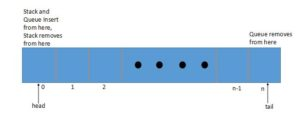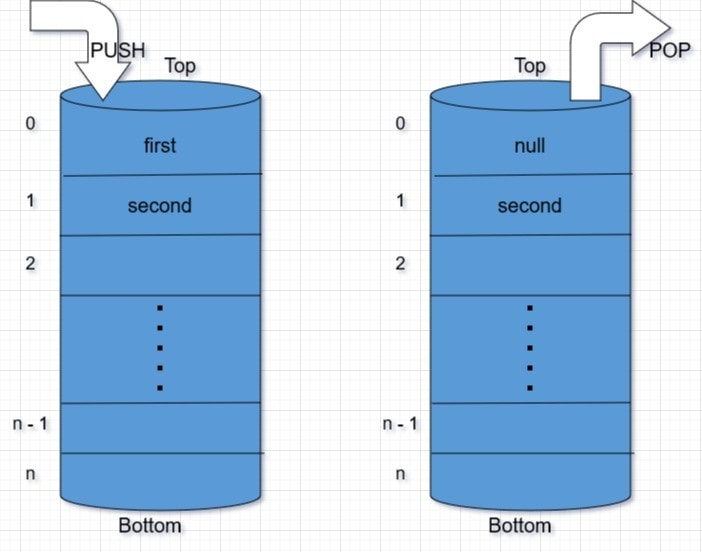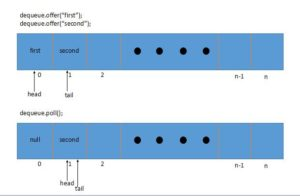## 1. Overview

In this tutorial, we’ll show how to use Java’s ArrayDeque class – which is an implementation of the Deque interface.

An ArrayDeque (also known as an “Array Double Ended Queue”, pronounced as “ArrayDeck”) is a special kind of a growable array that allows us to add or remove an element from both sides.

An ArrayDeque implementation can be used as a Stack (Last-In-First-Out) or a Queue(First-In-First-Out).

## 2. The API at a Glance

For each operation, we basically have two options.

The first group consists of methods that throw an exception if the operation fails. The other group returns a status or a value:

 Operation Method Method throwing Exception Insertion from Head offerFirst(e) addFirst(e) Removal from Head pollFirst() removeFirst() Retrieval from Head peekFirst() getFirst() Insertion from Tail offerLast(e) addLast(e) Removal from Tail pollLast() removeLast() Retrieval from Tail peekLast() getLast()

## 3. Using Methods

Let’s look at a few simple examples of how we can make use of the ArrayDeque.

### 3.1. Using ArrayDeque as a Stack

We’ll start with an example of how we can treat the class as a Stack – and push an element:

``````@Test
Deque<String> stack = new ArrayDeque<>();
stack.push("first");
stack.push("second");

assertEquals("second", stack.getFirst());
}
``````

Let’s also see how we can pop an element from the ArrayDeque – when used as a Stack:

``````@Test
public void whenPop_removesLast() {
Deque<String> stack = new ArrayDeque<>();
stack.push("first");
stack.push("second");

assertEquals("second", stack.pop());
}
``````

The pop method throws NoSuchElementException when a stack is empty.

### 3.2. Using ArrayDeque as a Queue

Let’s now start with a simple example showing how we can offer an element in an ArrayDeque – when used as a simple Queue:

``````@Test
Deque<String> queue = new ArrayDeque<>();
queue.offer("first");
queue.offer("second");

assertEquals("second", queue.getLast());
}
``````

And let’s see how we can poll an element from an ArrayDeque, also when used as a Queue:

``````@Test
public void whenPoll_removesFirst() {
Deque<String> queue = new ArrayDeque<>();
queue.offer("first");
queue.offer("second");

assertEquals("first", queue.poll());
}
``````

The poll method returns a null value if a queue is empty.

## 4. How’s ArrayDeque ImplementedUnder the hood, the ArrayDeque is backed by an array which doubles its size when it gets filled.

Initially, the array is initialized with a size of 16. It’s implemented as a double-ended queue where it maintains two pointers, namely a head and a tail.

Let’s see this logic in action – at a high level.

### 4.1. ArrayDeque as StackAs can be seen, when a user adds in an element using the push method, it moves the head pointer by one.

When we pop an element, it sets the element at the head position as null so the element could be garbage collected and then moves back the head pointer by one.

### 4.2. ArrayDeque as a QueueWhen we add in an element using the offer method, it moves the tail pointer by one.

When the user polls an element, it sets the element at the head position to null so the element can be garbage collected and then moves the head pointer.

### 4.3. Notes on ArrayDeque

• Null elements are not accepted
• Works significantly faster than the synchronized Stack
• It is a faster queue than LinkedList due to the better locality of reference
• Most operations have amortized constant time complexity
• An Iterator returned by an ArrayDeque is fail-fast
• ArrayDeque automatically doubles the size of an array when the head and tail pointer meets each other while adding an element

## 5. Conclusion

In this short article, we illustrated the usage of methods in ArrayDeque.

The implementation of all these examples can be found in the GitHub project; this is a Maven-based project, so it should be easy to import and run as is.

### Get started with Spring 5 and Spring Boot 2, through the Learn Spring course:

>> CHECK OUT THE COURSE